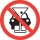# Down syndrome

Down syndrome is one of the serious diseases caused by a gene mutation. Down syndrome occurs in approximately every 550-born child. Express the incidence of Down's syndrome in newborns at per mille.

Result

p =  1.818

#### Solution:Leave us a comment of example and its solution (i.e. if it is still somewhat unclear...):

Showing 0 comments:Be the first to comment!#### To solve this example are needed these knowledge from mathematics:

Our permille calculator will help you quickly calculate various typical tasks with permilles.

## Next similar examples:

1. Apples 2James has 13 apples. He has 30 percent more apples than Sam. How many apples has Sam?
2. TVsProduction of television sets increased from 3,500 units to 4,200 units. Calculate the percentage of production increase.
3. Base, percents, valueBase is 344084 which is 100 %. How many percent is 384177?
4. New refrigeratorNew refrigerator sells for 1024 USD, Monday will be 25% discount. How much USD will save, and what will be the price?
5. ClassIn a class are 32 pupils. Of these are 8 boys. What percentage of girls are in the class?
6. GlovesI have a box with two hundred pieces of gloves in total, split into ten parcels of twenty pieces, and I sell three parcels. What percent of the total amount I sold?
7. Unknown numberIdentify unknown number which 1/5 is 40 greater than one tenth of that number.
8. InequationSolve the inequation: 5k - (7k - 1)≤ 2/5 . (5-k)-2
9. Percents - easyHow many percent is 432 out of 434?
10. EquationSolve the equation: 1/2-2/8 = 1/10; Write the result as a decimal number.
11. Sales offGoods is worth € 70 and the price of goods fell two weeks in a row by 10%. How many % decreased overall?
12. NumberWhat number is 20 % smaller than the number 198?
13. MO 2016 Numerical axisCat's school use a special numerical axis. The distance between the numbers 1 and 2 is 1 cm, the distance between the numbers 2 and 3 is 3 cm, between the numbers 3 and 4 is 5 cm and so on, the distance between the next pair of natural numbers is always in
14. Simplify 2Simplify expression: 5ab-7+3ba-9
15. Conference148 is the total number of employees. The conference was attended by 22 employees. How much is it in percent?
16. The percentages in practiceIf every tenth apple on the tree is rotten it can be expressed by percentages: 10% of the apples on the tree is rotten. Tell percent using the following information: a. in June rained 6 days b, increase worker pay 500 euros to 50 euros c, grabbed 21 fro
17. Car crashAfter a traffic accident, police measured 1.16 permille of alcohol in the driver's blood. What amount of alcohol did the driver have in the blood when about 5 kg of blood circulates in the human body?